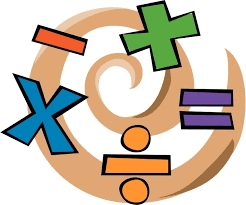# PLUS MINUS product

A. Deduct 15.728 from the sum of 23.234 and 6.23

B. Add 15.756 to the difference of 26.845 and 4.342

C. Find the product of 0.86 x 0.05

D. Multiply 5.234 by 4.45

E What is the product of 23.97 multiplied by 6.36?

A =  13.736
B =  38.259
C =  0.043
D =  23.2913
E =  152.4492

### Step-by-step explanation:Did you find an error or inaccuracy? Feel free to write us. Thank you!